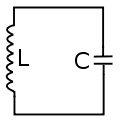# LC Oscillations seem impossible to meCurrent increases up to a certain point, and then decreases until it reaches zero. Then it does the same thing in the other direction. Here's my problem.

Without the inductor, the current would not increase over time: it would decay until the two plates had equal charge. With the inductor, I believe the current should decay even faster because inductors tend to create a magnetic flux that opposes the current change. Where is the current increase coming from?

Please be nice: I'm studying physics on my own for pleasure, and haven't taken classes.

AlephZero
Homework Helper
First think about a CR cicruit (replace your inductor by a resistor).

Suppose there is a switch in the circuit, so you can start with the capacitor fully charged and then close the switch.

When you close the switch the current instantly rises to at its maximum value, and decays to zero as the voltage across the capacitor reduces.

Now, think about the LC circut, again starting with the capacitor fully charged and closing the switch.

This time, the current can not "instantly" rise to its maximum value, because the back EMF generated in the inductor opposes it (Lenz's law). The back EMF depends on the rate of change of the current, not the magnitude of the current.

So, the current starts to increase gradually, and as the voltage across the capacitor decreases the rate of increase slows down because it takes less back EMF to oppose the incease.

Eventially, voltage across the capacitor has reduced to zero but the current has built up to its maximum value. At this point, all the energy in the circuit is stored in the magetic field of the inductor.

The current then decays back to zero, which produces a "back EMF" of the opposite sign in the inductor. When the current reaches 0, the voltage across the capacitor is equal to the starting voltage, but with the opposite sign.

The process then repeats with the currents and voltages reversed, to complete one cycle of the oscillation.

Hope that helps understand what it going on in a "non-mathematica" way.

That's perfect! Thank you. :D

Another question, though.

I think what's giving me trouble is where the back EMF is coming from. I had assumed that the motion of the electrons in the coil, moving orthogonally to those in the straight wire, caused a magnetic force on those electrons who have yet to enter the coil, slowing them down and thus decreasing the current.

But magnetic force can't change a charged particle's acceleration, it can only deflect it. What, then, causes the EMF?

That is, why does induction happen?

davenn
Gold Member
AlephZero
Homework Helper
There are two different ways to think about electrical circuits.

The "engineering" way is in terms of resistance, capacitance, inductance, current, voltage, etc.

The "physics" way is in terms of electromagnetic fields and the movement of charged particles, and even "simple" properties like resistance also involve quantum mechanics.

Trying to mix up the two approaches is a very good way to get confused. You are right that Lenz's law is a consequence of Maxwell's equations, but at the "beginning circuit analysis in electrical engineering" level it is easier just to treat it as an experimental fact about inductors.

Of course if you are analysing a high frequency circuit, you can't ignore the fact that every part of the circuit (including the connecting wires) creates an EM field and therefore acts both as an inductor and as a capacitor - but that's not "beginning circuit analysis".

The "physics" way is in terms of electromagnetic fields and the movement of charged particles, and even "simple" properties like resistance also involve quantum mechanics.

This is the way I was trying to understand it, by using Biot-Savart. But it doesn't really work, since it can't explain how a increase in magnetic force causes a decrease in current--magnetic fields can't decelerate charged particles, as I mentioned.

I will just think of it experimentally, as you said. Hopefully when I get to quantum mechanics it will work itself out. Thank you.